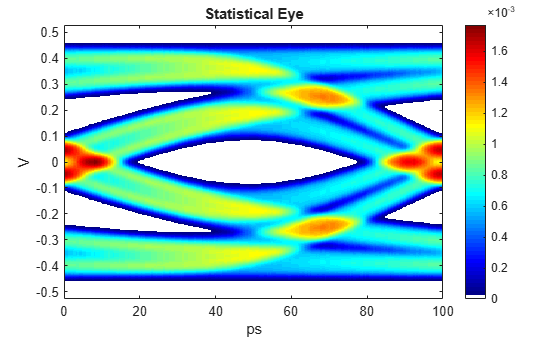pulse2stateye

Statistical eye from pulse response

Description

example

[S,VH,TH] = pulse2stateye(P,N,M) calculates and plots the statistical eye S from a pulse response P with N samples per symbol and M levels of modulation.

The statistical eye is generated by progressively accumulating the histogram of each vertical slice VH by perturbing the ideal cursor voltage by the inter-symbol interference (ISI) voltages defined by the pulse response.

[___,S1] = pulse2stateye(P,N,M) also returns the symbol transition histograms and crosstalk histogram S1.

pulse2stateye(P,N,M) calculates and plots the statistical eye to the current figure.

Examples

collapse all

Load the pulse response column matrix from a file.

modulation = 2;

Calculate the statistical eye.

[stateye,vh,th] = pulse2stateye(pulse,SamplesPerSymbol,modulation);

Visualize the statistical eye using a color map.

cmap = serdes.utilities.SignalIntegrityColorMap;
figure,
imagesc(th*SymbolTime*1e12,vh,stateye)
colormap(cmap)
colorbar
axis('xy')
xlabel('ps')
ylabel('V')
title('Statistical Eye')Input Arguments

collapse all

Input pulse response, specified as a column matrix. The first column contains the primary pulse response and the subsequent columns (if any) contain the crosstalk pulse responses.

Data Types: double

Number of samples per symbol, specified as a positive integer scalar.

Data Types: double

Number of modulation levels, specified as a positive integer scalar. M defines the modulation scheme used in the statistical eye calculation.

• If M = 2, the modulation scheme is nonreturn to zero (NRZ).

• If M = 4, the modulation scheme is four-level pulse amplitude modulation (PAM4).

Data Types: double

Output Arguments

collapse all

Statistical eye of the pulse response P, returned as a matrix.

Data Types: double

Vertical voltage histogram bin centers, returned as a vector.

Data Types: double

Horizontal time histogram bin centers, returned as a vector.

Data Types: double

Symbol transition histograms and crosstalk histogram, returned as a 3-D matrix.S1 is used to calculate the statistical eye S.

Data Types: double